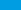# Math Formulas$$x = x^2$$

you can write formula inline : $$x = x^2$$ this way.